## Fixed and random effects models

• When you have repeated observations per individual this is a problem and an advantage:
• the observations are not independent
• we can use the repetition to get better parameter estimates
• If we pooled the observations and used e.g., OLS we would have biased estimates
• If we fit fixed-effect or random-effect models which take account of the repetition we can control for fixed or random individual differences.

• In the econometrics literature these models are called cross-sectional time-series' models, because we have time-series of observations at individual rather than aggregate level.
• If we have a small number of individuals, we can simply fit a dummy for the individual: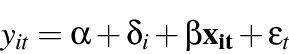• This can be considered a fixed-effects' model because the regression line is raised or lowered by a fixed amount for each individual
• If there are many individuals this cannot be done directly, but there are mathematically equivalent models which achieve the same effect
• This model is appropriate where we consider each individual to have a fixed effect shifting the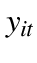up or down
• We may prefer to consider the individual differences as random disturbances drawn from some specified distribution: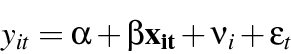• This has the advantage of using fewer degrees of freedom, and that individual differences are considered random rather than fixed and estimable.
• It has the disadvantage of requiring no correlation between the regressors (the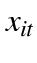s) and the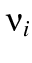: there are tests for this assumption (Hausman test).

The xt series of commands provide tools for analyzing cross-sectional time-
series (panel) datasets:
help xtdes      Describe pattern of xt data
help xtsum      Summarize xt data
help xttab      Tabulate xt data
help xtreg      Fixed-, between- and random-effects, and population-
averaged linear models
help xtdata     Faster specification searches with xt data
help xtlogit    Fixed-effects, random-effects, & population-averaged
logit models
help xtprobit   Random-effects and population-averaged probit models
help xttobit    Random-effects tobit models
help xtpois     Fixed-effects, random-effects, & population-averaged
Poisson models
help xtnbreg    Fixed-effects, random-effects, & population-averaged
negative binomial models
help xtclog     Random-effects and population-averaged cloglog models
help xtintreg   Random-effects interval data regression models
help xtrchh     Hildreth-Houck random coefficients models
help xtgls      Panel-data models using GLS
help xtgee      Population-averaged panel-data models using GEE


• Fitting these models in Stata is easy:
• With data in long format, one record per individual per wave
• . xtreg yvar x1 x2, fe i(pid)
• . xtreg yvar x1 x2, re i(pid)
• . xtlogit yvar x1 x2, re i(pid)
• Reference: William Greene, Econometric Analysis, Maxwell Macmillan 1991, Ch 16 section 4.© Brendan Halpin (e-mail) 23-Apr-2012 Department of Sociology, University of Limerick Taught programme: MA in Sociology (Applied Social Research), Short course, May 14/15 2012: Categorical Data Analysis for Social Scientists